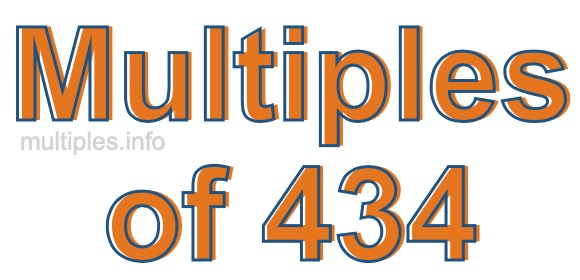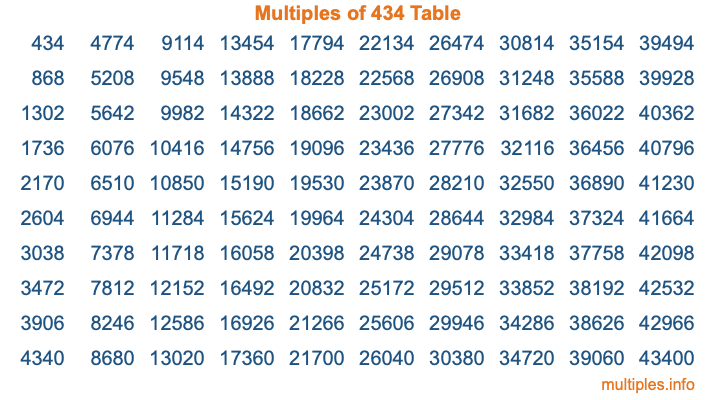Multiples of 434Welcome to the Multiples of 434 page. Here we will first teach you everything you will ever need to know about the multiples of 434, and then give you a study guide summary of everything we taught you to make sure you remember it all. Use this page to look up facts and learn information about the multiples of 434. This page will make you a multiples of four hundred thirty-four expert!

Definition of Multiples of 434
Multiples of 434 are all the numbers that when divided by 434 equal an integer. Each of the multiples of 434 are called a multiple. A multiple of 434 is created by multiplying 434 by an integer.

Therefore, to create a list of multiples of 434, you start with 1 multiplied by 434, then 2 multiplied by 434, then 3 multiplied by 434, and so on for as long as you want. Thus, the list of the first five multiples of 434 is 434, 868, 1302, 1736, and 2170. To see a larger list of multiples of 434, see the printable image of Multiples of 434 further down on this page. We also have a category where you can choose any nth multiple of 434.

Multiples of 434 Checker
The Multiples of 434 Checker below checks to see if any number of your choice is a multiple of 434. In other words, it checks to see if there is any number (integer) that when multiplied by 434 will equal your number. To do that, we divide your number by 434. If the the quotient is an integer, then your number is a multiple of 434.

Is  a multiple of 434?

Least Common Multiple of 434 and ...
A Least Common Multiple (LCM) is the lowest multiple that two or more numbers have in common. This is also called the smallest common multiple or lowest common multiple and is useful to know when you are adding our subtracting fractions. Enter one or more numbers below (434 is already entered) to find the LCM.

Check out our LCM Calculator if you need more details about the Least Common Multiple or if you need the LCM for different numbers for adding and subtraction fractions.

nth Multiple of 434
As we stated above, 434 is the first multiple of 434, 868 is the second multiple of 434, 1302 is the third multiple of 434, and so on. Enter a number below to find the nth multiple of 434.

th multiple of 434

Multiples of 434 vs Factors of 434
434 is a multiple of 434 and a factor of 434, but that is where the similarities end. All postive multiples of 434 are 434 or greater than 434. All positive factors of 434 are 434 or less than 434.

Below is the beginning list of multiples of 434 and the factors of 434 so you can compare:

Multiples of 434: 434, 868, 1302, 1736, 2170, etc.

Factors of 434: 1, 2, 7, 14, 31, 62, 217, 434

As you can see, the multiples of 434 are all the numbers that you can divide by 434 to get a whole number. The factors of 434, on the other hand, are all the whole numbers that you can multiply by another whole number to get 434.

It's also interesting to note that if a number (x) is a factor of 434, then 434 will also be a multiple of that number (x).

Multiples of 434 vs Divisors of 434
The divisors of 434 are all the integers that 434 can be divided by evenly. Below is a list of the divisors of 434.

Divisors of 434: 1, 2, 7, 14, 31, 62, 217, 434

The interesting thing to note here is that if you take any multiple of 434 and divide it by a divisor of 434, you will see that the quotient is an integer.

Multiples of 434 Table
Below is an image of the first 100 multiples of 434 in a table. The table is in chronological order, column by column. The first column has the first ten multiples of 434, the second column has the next ten multiples of 434, and so on.The Multiples of 434 Table is also referred to as the 434 Times Table or Times Table of 434. You are welcome to print out our table for your studies.

Negative Multiples of 434
Although not often discussed or needed in math, it is worth mentioning that you can make a list of negative multiples of 434 by multiplying 434 by -1, then by -2, then by -3, and so on, to get the following list of negative multiples of 434:

-434, -868, -1302, -1736, -2170, etc.

Multiples of 434 Summary
Below is a summary of important Multiples of 434 facts that we have discussed on this page. To retain the knowledge on this page, we recommend that you read through the summary and explain to yourself or a study partner why they hold true.

There are an infinite number of multiples of 434.

A multiple of 434 divided by 434 will equal a whole number.

434 divided by a factor of 434 equals a divisor of 434.

The nth multiple of 434 is n times 434.

The largest factor of 434 is equal to the first positive multiple of 434.

434 is a multiple of every factor of 434.

434 is a multiple of 434.

A multiple of 434 divided by a divisor of 434 equals an integer.

434 divided by a divisor of 434 equals a factor of 434.

Any integer times 434 will equal a multiple of 434.

Multiples of a Number
Here you can get the multiples of another number, all with the same attention to detail as we did for multiples of 434 on this page.

Multiples of
Multiples of 435
Did you find our page about multiples of four hundred thirty-four educational? Do you want more knowledge? Check out the multiples of the next number on our list!